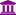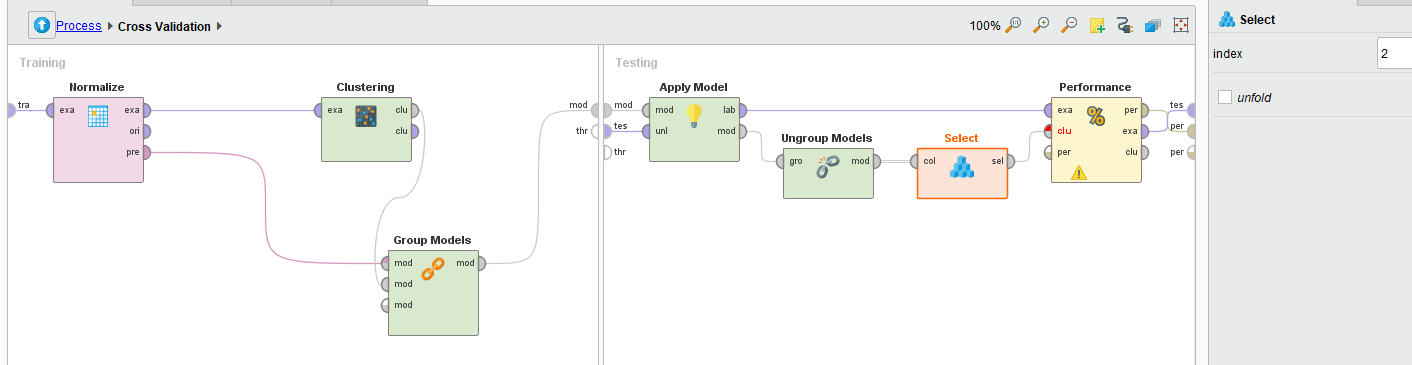# Normalization (training) with clustering (group model) does not work as expected

Member, University Professor Posts: 19University Professor
edited July 9 in Help
Using a Normalization operator alongside k-Means operator to create a group model within a Cross-Validation or Split-Validation does not work because the Performance (Cluster Distance Performance) operator expects a CentroidClusterModel but instead received a GroupedModel. It seems that the Performance (Cluster Distance Performance) operator needs to be updated to accommodate a grouped model.
A simple example using the Iris dataset in the RapidMiner Samples directory is attached showing the issue.

Tagged:

• Employee, RapidMiner Certified Analyst, RapidMiner Certified Expert, Member Posts: 195RM Data Scientist
edited July 12
Dear Prof @amitdeokar

Thanks for sharing the process of cross validated K-means. The normalize pre-processing model is grouped with clustering model in the training phase. But the clustering performance operator can only take a cluster model as a input, not a grouped model.```<?xml version="1.0" encoding="UTF-8"?><process version="9.3.001">
<context>
<input/>
<output/>
<macros/>
</context>
<operator activated="true" class="process" compatibility="9.3.001" expanded="true" name="Process">
<parameter key="logverbosity" value="init"/>
<parameter key="random_seed" value="2001"/>
<parameter key="send_mail" value="never"/>
<parameter key="process_duration_for_mail" value="30"/>
<parameter key="encoding" value="SYSTEM"/>
<process expanded="true">
<operator activated="true" class="retrieve" compatibility="9.3.001" expanded="true" height="68" name="Retrieve Iris (2)" width="90" x="45" y="34">
<parameter key="repository_entry" value="//Samples/data/Iris"/>
</operator>
<operator activated="true" class="concurrency:cross_validation" compatibility="9.3.001" expanded="true" height="145" name="Cross Validation" width="90" x="313" y="34">
<parameter key="split_on_batch_attribute" value="false"/>
<parameter key="leave_one_out" value="false"/>
<parameter key="number_of_folds" value="10"/>
<parameter key="sampling_type" value="automatic"/>
<parameter key="use_local_random_seed" value="false"/>
<parameter key="local_random_seed" value="1992"/>
<parameter key="enable_parallel_execution" value="true"/>
<process expanded="true">
<operator activated="true" class="normalize" compatibility="9.3.001" expanded="true" height="103" name="Normalize" width="90" x="45" y="34">
<parameter key="return_preprocessing_model" value="false"/>
<parameter key="create_view" value="false"/>
<parameter key="attribute_filter_type" value="all"/>
<parameter key="attribute" value=""/>
<parameter key="attributes" value=""/>
<parameter key="use_except_expression" value="false"/>
<parameter key="value_type" value="numeric"/>
<parameter key="use_value_type_exception" value="false"/>
<parameter key="except_value_type" value="real"/>
<parameter key="block_type" value="value_series"/>
<parameter key="use_block_type_exception" value="false"/>
<parameter key="except_block_type" value="value_series_end"/>
<parameter key="invert_selection" value="false"/>
<parameter key="include_special_attributes" value="false"/>
<parameter key="method" value="Z-transformation"/>
<parameter key="min" value="0.0"/>
<parameter key="max" value="1.0"/>
<parameter key="allow_negative_values" value="false"/>
</operator>
<operator activated="true" class="concurrency:k_means" compatibility="9.3.001" expanded="true" height="82" name="Clustering" width="90" x="313" y="34">
<parameter key="remove_unlabeled" value="false"/>
<parameter key="k" value="5"/>
<parameter key="max_runs" value="10"/>
<parameter key="determine_good_start_values" value="true"/>
<parameter key="measure_types" value="BregmanDivergences"/>
<parameter key="mixed_measure" value="MixedEuclideanDistance"/>
<parameter key="nominal_measure" value="NominalDistance"/>
<parameter key="numerical_measure" value="EuclideanDistance"/>
<parameter key="divergence" value="SquaredEuclideanDistance"/>
<parameter key="kernel_gamma" value="1.0"/>
<parameter key="kernel_sigma1" value="1.0"/>
<parameter key="kernel_sigma2" value="0.0"/>
<parameter key="kernel_sigma3" value="2.0"/>
<parameter key="kernel_degree" value="3.0"/>
<parameter key="kernel_shift" value="1.0"/>
<parameter key="kernel_a" value="1.0"/>
<parameter key="kernel_b" value="0.0"/>
<parameter key="max_optimization_steps" value="100"/>
<parameter key="use_local_random_seed" value="false"/>
<parameter key="local_random_seed" value="1992"/>
</operator>
<operator activated="true" class="group_models" compatibility="9.3.001" expanded="true" height="103" name="Group Models" width="90" x="380" y="187"/>
<connect from_port="training set" to_op="Normalize" to_port="example set input"/>
<connect from_op="Normalize" from_port="example set output" to_op="Clustering" to_port="example set"/>
<connect from_op="Normalize" from_port="preprocessing model" to_op="Group Models" to_port="models in 1"/>
<connect from_op="Clustering" from_port="cluster model" to_op="Group Models" to_port="models in 2"/>
<connect from_op="Group Models" from_port="model out" to_port="model"/>
<portSpacing port="source_training set" spacing="0"/>
<portSpacing port="sink_model" spacing="0"/>
<portSpacing port="sink_through 1" spacing="0"/>
</process>
<process expanded="true">
<operator activated="true" class="apply_model" compatibility="9.3.001" expanded="true" height="82" name="Apply Model" width="90" x="45" y="34">
<list key="application_parameters"/>
<parameter key="create_view" value="false"/>
</operator>
<operator activated="true" class="ungroup_models" compatibility="9.3.001" expanded="true" height="68" name="Ungroup Models" width="90" x="179" y="85"/>
<operator activated="true" class="select" compatibility="9.3.001" expanded="true" height="68" name="Select" width="90" x="313" y="85">
<parameter key="index" value="2"/>
<parameter key="unfold" value="false"/>
</operator>
<operator activated="true" class="cluster_distance_performance" compatibility="9.3.001" expanded="true" height="103" name="Performance" width="90" x="447" y="34">
<parameter key="main_criterion" value="Avg. within centroid distance"/>
<parameter key="main_criterion_only" value="false"/>
<parameter key="normalize" value="false"/>
<parameter key="maximize" value="false"/>
</operator>
<connect from_port="model" to_op="Apply Model" to_port="model"/>
<connect from_port="test set" to_op="Apply Model" to_port="unlabelled data"/>
<connect from_op="Apply Model" from_port="labelled data" to_op="Performance" to_port="example set"/>
<connect from_op="Apply Model" from_port="model" to_op="Ungroup Models" to_port="grouped model"/>
<connect from_op="Ungroup Models" from_port="models" to_op="Select" to_port="collection"/>
<connect from_op="Select" from_port="selected" to_op="Performance" to_port="cluster model"/>
<connect from_op="Performance" from_port="performance" to_port="performance 1"/>
<connect from_op="Performance" from_port="example set" to_port="test set results"/>
<portSpacing port="source_model" spacing="0"/>
<portSpacing port="source_test set" spacing="0"/>
<portSpacing port="source_through 1" spacing="0"/>
<portSpacing port="sink_test set results" spacing="0"/>
<portSpacing port="sink_performance 1" spacing="0"/>
<portSpacing port="sink_performance 2" spacing="0"/>
</process>
</operator>
<connect from_op="Retrieve Iris (2)" from_port="output" to_op="Cross Validation" to_port="example set"/>
<connect from_op="Cross Validation" from_port="model" to_port="result 1"/>
<connect from_op="Cross Validation" from_port="performance 1" to_port="result 2"/>
<portSpacing port="source_input 1" spacing="0"/>
<portSpacing port="sink_result 1" spacing="21"/>
<portSpacing port="sink_result 2" spacing="0"/>
<portSpacing port="sink_result 3" spacing="252"/>
</process>
</operator>
</process>
```

Best,

YY
• Moderator, RapidMiner Certified Analyst, RapidMiner Certified Expert, Member Posts: 1,178Unicorn
Another solution in similar types of scenarios would be to normalize your data outside the cross validation rather than inside on the training set.  This removes the need to pass the normalization model through to the test set so you don't need group models at all.  While this is not the preferred setup, because this technically leaks information from the full dataset into the training data, the effect is probably very small (you can actually do it both ways to see how large the effect is and whether it is a concern with your particular datdaset).
Brian T.
Lindon Ventures
Data Science Consulting from Certified RapidMiner Experts